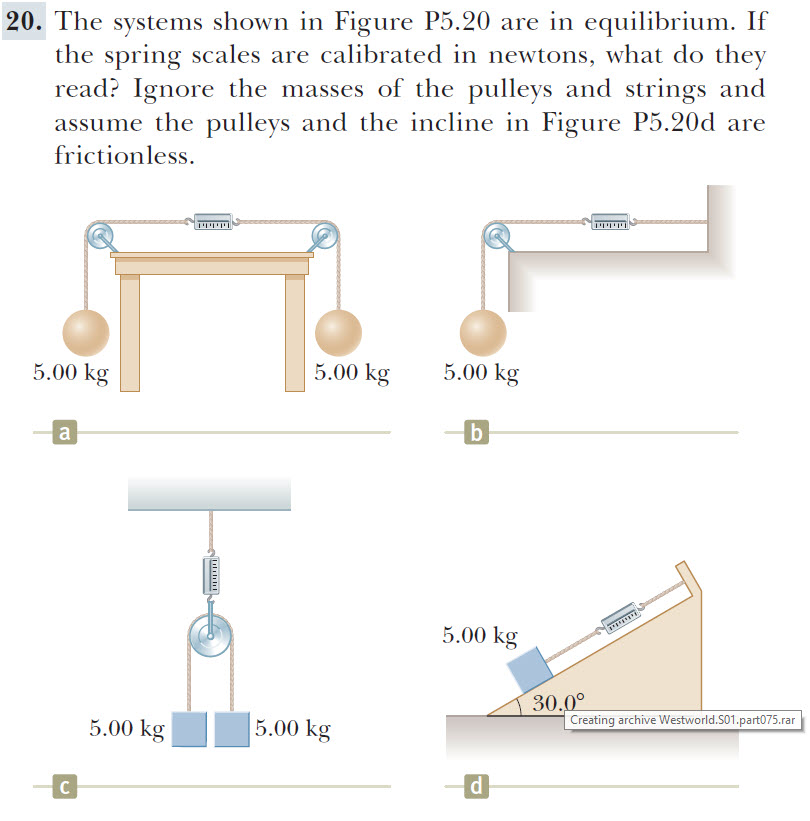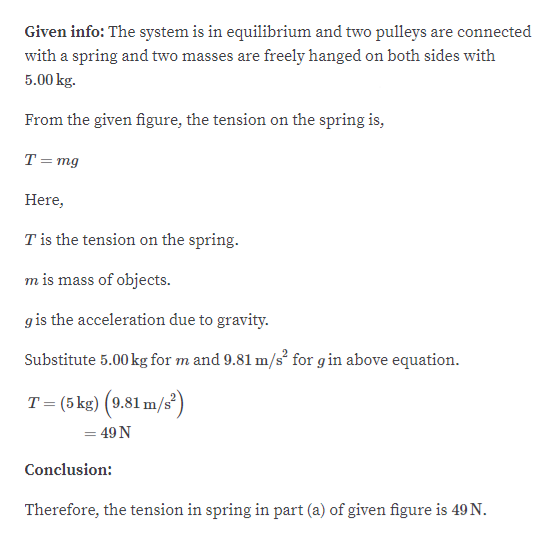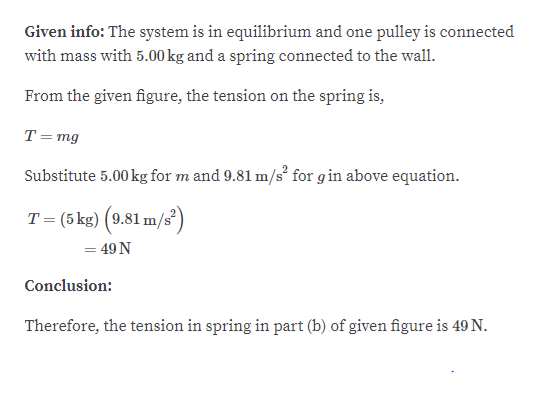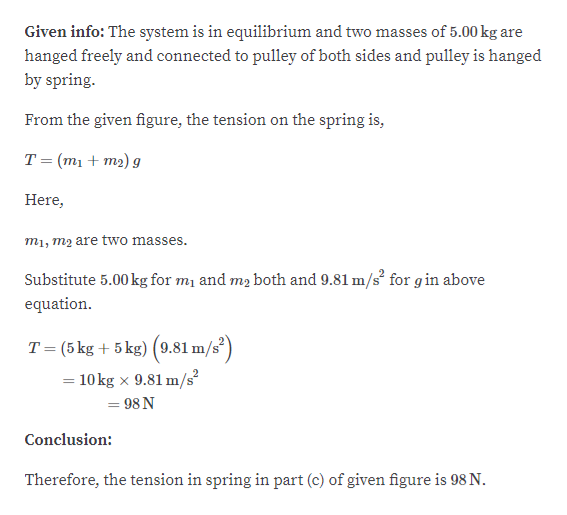# 20. The systems shown in Figure P5.20 are in equilibrium. Ifthe spring scales are calibrated in newtons, what do theyread? Ignore the masses of the pulleys and strings andassume the pulleys and the incline in Figure P5.20d arefrictionless.5.00 kg5.00 kg5.00 kgb5.00 kg30.00Creating archive Westworld.S01 .part075. rar5.00 kg5.00 kgсra

Question
39 viewshelp_outlineImage Transcriptionclose20. The systems shown in Figure P5.20 are in equilibrium. If the spring scales are calibrated in newtons, what do they read? Ignore the masses of the pulleys and strings and assume the pulleys and the incline in Figure P5.20d are frictionless. 5.00 kg 5.00 kg 5.00 kg b 5.00 kg 30.00 Creating archive Westworld.S01 .part075. rar 5.00 kg 5.00 kg с ra fullscreen
check_circle

Step 1

part Ahelp_outlineImage TranscriptioncloseGiven info: The system is in equilibrium and two pulleys are connected with a spring and two masses are freely hanged on both sides with 5.00 kg From the given figure, the tension on the spring is, T =mg Here T is the tension on the spring. m is mass of objects g is the acceleration due to gravity Substitute 5.00kg for m and 9.81 m/s2 for gin above equation T (5 kg) (9.81 m/s) = 49 N Conclusion Therefore, the tension in spring in part (a) of given figure is 49 N. fullscreen
Step 2

Part Bhelp_outlineImage TranscriptioncloseGiven info: The system is in equilibrium and one pulley is connected with mass with 5.00 kg and a spring connected to the wall From the given figure, the tension on the spring is, T = mg Substitute 5.00kg for m and 9.81 m/s for gin above equation T (5 kg) (9.81 m/s = 49 N Conclusion: Therefore, the tension in spring in part (b) of given figure is 49 N. fullscreen
Step 3

Part C :...help_outlineImage TranscriptioncloseGiven info: The system is in equilibrium and two masses of 5.00 kg are hanged freely and connected to pulley of both sides and pulley is hanged by spring. From the given figure, the tension on the spring is, T (m1m2) g Here mi, m2 are two masses. Substitute 5.00 kg for mi and m2 both and 9.81 m/s for gin above equation T-(5 kg + 5kg) (9.81 m/s?) 10kg x 9.81 m/ 98 N Conclusion: Therefore, the tension in spring in part (c) of given figure is 98 N. fullscreen

### Want to see the full answer?

See Solution

#### Want to see this answer and more?

Solutions are written by subject experts who are available 24/7. Questions are typically answered within 1 hour.*

See Solution
*Response times may vary by subject and question.
Tagged in

### Thermodynamics